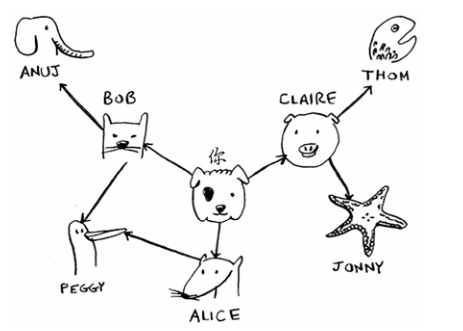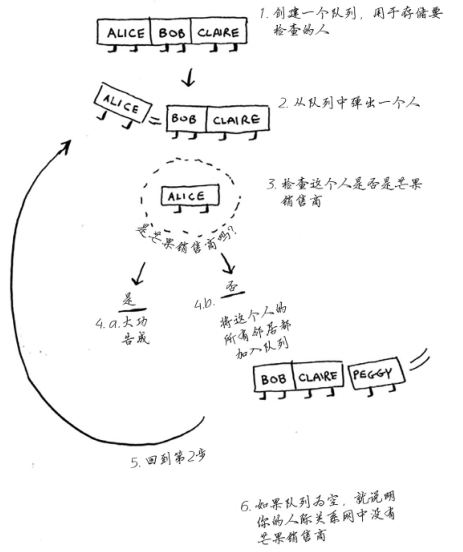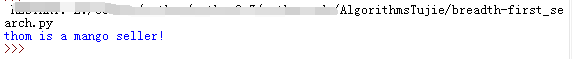﻿ 《算法图解》第六章笔记_广度优先搜索

## 一、算法描述

• 第一类问题：从节点A出发，有前往节点B的路径吗？（在你的人际关系网中，有芒果销售商吗？）
• 第二类问题：从节点A出发，前往节点B的哪条路径最短？（哪个芒果销售商与你的关系最近？）

## 二、实现图```graph = {}
graph["you"] = ["alice", "bob", "claire"]
graph["bob"] = ["anuj", "peggy"]
graph["alice"] = ["peggy"]
graph["claire"] = ["thom", "jonny"]
graph["anuj"] = []
graph["peggy"] = []
graph["thom"] = []
graph["jonny"] = []```

## 三、实现算法```from collections import deque

def person_is_seller(name):
return name[-1] == 'm'

graph = {}
graph["you"] = ["alice", "bob", "claire"]
graph["bob"] = ["anuj", "peggy"]
graph["alice"] = ["peggy"]
graph["claire"] = ["thom", "jonny"]
graph["anuj"] = []
graph["peggy"] = []
graph["thom"] = []
graph["jonny"] = []

def search(name):
search_queue = deque() # 创建一个队列
search_queue += graph[name] # 将你的邻居都加入到这个搜索队列中
searched = [] # 该数组用于记录检查过的人
while search_queue: # 只要队列不为空
person = search_queue.popleft() # 就取出其中的第一个人
if not person in searched: # 仅当这个人没检查过时才检查
if person_is_seller(person): # 检查这个人是否是芒果销售商
print (person + " is a mango seller!") # 是芒果销售商
return True
else:
search_queue += graph[person] # 不是芒果销售商。将这个人的朋友都加入搜索队列
searched.append(person) # 将这个人标记为检查过
return False # 队列中没人是芒果销售商

search("you")```## 四、小结

• 队列是先进先出的。
• 栈是后进先出的。
• 你需要按加入顺序检查搜索列表中的人，否则找到的就不是最短路径，因此搜索列表必须是队列。
• 对于检查过的人，务必不要再去检查，否则可能导致无限循环。